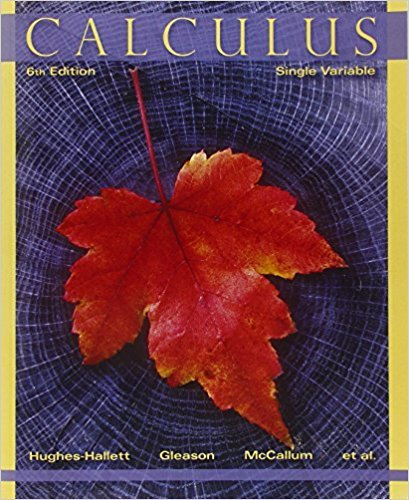×
×

# Solutions for Chapter 10.4: THE ERROR IN TAYLOR POLYNOMIAL APPROXIMATIONS## Full solutions for Calculus: Single Variable | 6th Edition

ISBN: 9780470888643Solutions for Chapter 10.4: THE ERROR IN TAYLOR POLYNOMIAL APPROXIMATIONS

Solutions for Chapter 10.4
4 5 0 334 Reviews
17
2
##### ISBN: 9780470888643

This textbook survival guide was created for the textbook: Calculus: Single Variable , edition: 6. Since 33 problems in chapter 10.4: THE ERROR IN TAYLOR POLYNOMIAL APPROXIMATIONS have been answered, more than 35505 students have viewed full step-by-step solutions from this chapter. This expansive textbook survival guide covers the following chapters and their solutions. Chapter 10.4: THE ERROR IN TAYLOR POLYNOMIAL APPROXIMATIONS includes 33 full step-by-step solutions. Calculus: Single Variable was written by and is associated to the ISBN: 9780470888643.

Key Calculus Terms and definitions covered in this textbook
• Commutative properties

a + b = b + a ab = ba

• Directed line segment

See Arrow.

• Heron’s formula

The area of ¢ABC with semiperimeter s is given by 2s1s - a21s - b21s - c2.

• Inverse cosine function

The function y = cos-1 x

• Limit to growth

See Logistic growth function.

• Linear combination of vectors u and v

An expression au + bv , where a and b are real numbers

• Origin

The number zero on a number line, or the point where the x- and y-axes cross in the Cartesian coordinate system, or the point where the x-, y-, and z-axes cross in Cartesian three-dimensional space

An angle in standard position whose terminal side lies on an axis.

A procedure for fitting a quadratic function to a set of data.

The measure of a central angle whose intercepted arc has a length equal to the circle’s radius.

The distance from a point on a circle (or a sphere) to the center of the circle (or the sphere).

• Real axis

See Complex plane.

• Reciprocal identity

An identity that equates a trigonometric function with the reciprocal of another trigonometricfunction.

• Relation

A set of ordered pairs of real numbers.

• Remainder theorem

If a polynomial f(x) is divided by x - c , the remainder is ƒ(c)

• Riemann sum

A sum where the interval is divided into n subintervals of equal length and is in the ith subinterval.

• Semiminor axis

The distance from the center of an ellipse to a point on the ellipse along a line perpendicular to the major axis.

• Slope-intercept form (of a line)

y = mx + b

• Sum of an infinite geometric series

Sn = a 1 - r , |r| 6 1

• Vertices of a hyperbola

The points where a hyperbola intersects the line containing its foci.

×